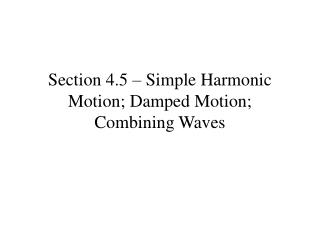DownloadDownload PresentationSection 4.5 – Simple Harmonic Motion; Damped Motion; Combining Waves

Section 4.5 – Simple Harmonic Motion; Damped Motion; Combining Waves

Download PresentationSection 4.5 – Simple Harmonic Motion; Damped Motion; Combining Waves

- - - - - - - - - - - - - - - - - - - - - - - - - - - E N D - - - - - - - - - - - - - - - - - - - - - - - - - - -
Presentation Transcript

1. Section 4.5 – Simple Harmonic Motion; Damped Motion; Combining Waves

2. Equilibrium is the location where the object is at rest A C B equilibrium

3. The amplitude of vibration is the distance from the equilibrium position to its point of greatest displacement (A or C). The period of a vibrating object is the time required to complete one vibration - that is, the time required to go from point A through B to C and back to A.

4. Simple harmonic motion is a special kind of vibrational motion in which the acceleration a of the object is directly proportional to the negative of its displacement d from its rest position. That is, a = -kd, k > 0.

5. Theorem Simple Harmonic Motion An object that moves on a coordinate axis so that its distance d from the origin at time t is given by either

6. The frequency f of an object in simple harmonic motion is the number of oscillations per unit of time. Thus,

7. Suppose that the distance d (in centimeters) an object travels in time t (in seconds) satisfies the equation (a) Describe the motion of the object. Simple harmonic (b) What is the maximum displacement from its resting position?

8. Suppose that the distance d (in centimeters) an object travels in time t (in seconds) satisfies the equation (c) What is the time required for one oscillation? (d) What is the frequency?

9. The simple harmonic motion on the previous slides assumes that there is no resistance to the motion…no friction. The motion of such systems will continue indefinitely In most physical systems, this is not the case. Friction or resistive forces remove energy from the system, dampening its motion.

10. Theorem Damped Motion The displacement d of an oscillating object from its at rest position at time t is given by where b is a damping factor (damping coefficient) and m is the mass of the oscillating object.

11. Suppose that a simple pendulum with a bob of mass 10 grams and a damping factor of .8 grams/sec is pulled 20 centimeters from its at rest position and released. The period of the pendulum without the damping effect is 4 seconds. 20 cm

12. Find the equation that describes the position of the pendulum bob. • Using a graphing utility, graph the function found in part (a). • Determine the maximum displacement of the bob after the first oscillation. • What happens to the displacement of the bob as time increases without bound?

13. Find the equation that describes the position of the pendulum bob. m = 10, a = 20, b = 0.8 The period under simple harmonic motion is 4 seconds.

14. c. Determine the maximum displacement of the bob after the first oscillation. About 17 cm

15. d. What happens to the displacement of the bob as time increases without bound? Notice that the dampened motion formula contains the factor What happens to this factor as t grows without bound, t -> ∞?

16. Read pages 305-307 on Combining Waves • To graph a function h(x) = f(x) + g(x): • Graph f(x) and g(x) • Select several x values from the domains of the f(x) and g(x) • Calculate f(x) and g(x) for the values of x selected in 2. • The value of h(x) for a given value of x will be the sum of f(x) and g(x).

17. Example: If we pick x = 2 and discover that for some given f(x) and g(x) we have f(2) = 4, and g(2) = 7, then the value of h(2) = 4 + 7 = 11, and the point (2,11) appears on the graph of h(x).